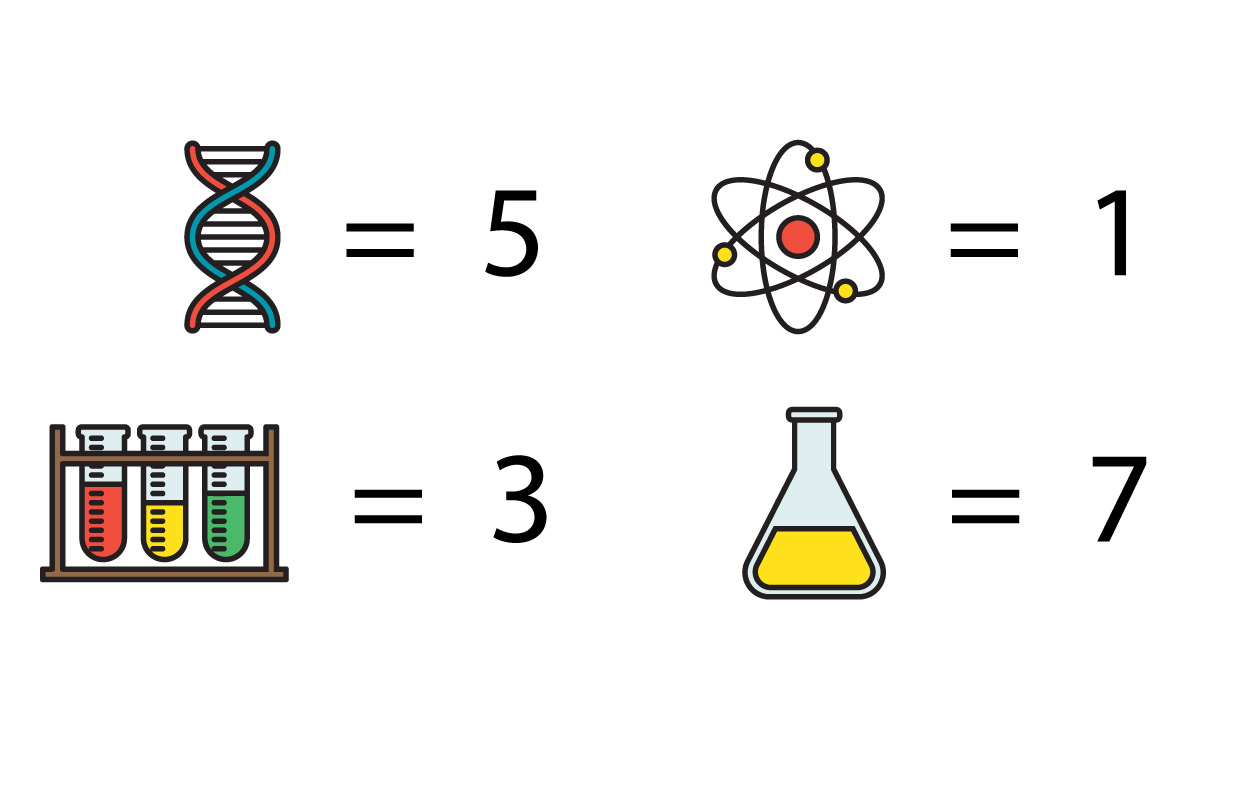# What's new## A strange solution brainteaser

By Jasmine, 1 July 2021 Brainteaser

Difficulty: Fun

Arafa was visiting a science centre to attend a chemistry workshop. She’d received a confirmation email that told her to head to the room number represented by a yellow flask.

Can you help Arafa find the right room number?Scroll down or click for a hint, or the answer!## Brainteaser hint

Looking more closely at the email, Arafa found a joke that might be a clue.Arafa needed to find room 7.

There are a few strategies that can be used to find the answer. Here’s one way of working it out:

• For the first line, 20 ÷ 4 (the number of DNA symbols) = 5. That means that each DNA symbol is a 5. You can check this by swapping the symbols for 5s: 5 + 5 + 5 + 5 = 20.
• For the second line you already know that the DNA symbol represents a 5. If you look at the answer 7 and subtract 5, 7 – 5 = 2. That means atom + atom = 2, and each atom is a 1. You can check this: 1 + 1 + 5 = 7.
• Next look at the third line. If you know what a square root is, you can use it to find the answer. Otherwise, you can simply try different numbers to try and work out what number the test tube rack is. You should find that 3 x 3 = 9, and the test tube rack is a 3.
• For the final line, you can first swap in all your previous answers and get 3 + 1 + 5 – ? = 2. That means 9 – ? = 2. So the yellow flask represents a 7. To check, we calculate 3 + 1 + 5 – 7 = 2. That means room 7 is the correct room number!

For more brainteasers and puzzles for kids, subscribe to Double Helix magazine!Categories: